Website Pembelajaran Pemrograman dan Info Game Terupdate

# Looping In Javascript Program

What will you do when told to print a sentence repeated?

For example:

"Please show the phrase "Learning Javascript in FajarYusuf.Com!on my website as much as 10 times "

Maybe you could write the function document.write () 10 times like this:

``````<!DOCTYPE html>
<html lang="en">
<title>looping</title>
<body>
<script>
document.write("<p>Learning Javascript in FajarYusuf.Com!</p>");
document.write("<p>Learning Javascript in FajarYusuf.Com!</p>");
document.write("<p>Learning Javascript in FajarYusuf.Com!</p>");
document.write("<p>Learning Javascript in FajarYusuf.Com!</p>");
document.write("<p>Learning Javascript in FajarYusuf.Com!</p>");
document.write("<p>Learning Javascript in FajarYusuf.Com!</p>");
document.write("<p>Learning Javascript in FajarYusuf.Com!</p>");
document.write("<p>Learning Javascript in FajarYusuf.Com!</p>");
document.write("<p>Learning Javascript in FajarYusuf.Com!</p>");
document.write("<p>Learning Javascript in FajarYusuf.Com!</p>");
</script>
</body>
</html>
```

```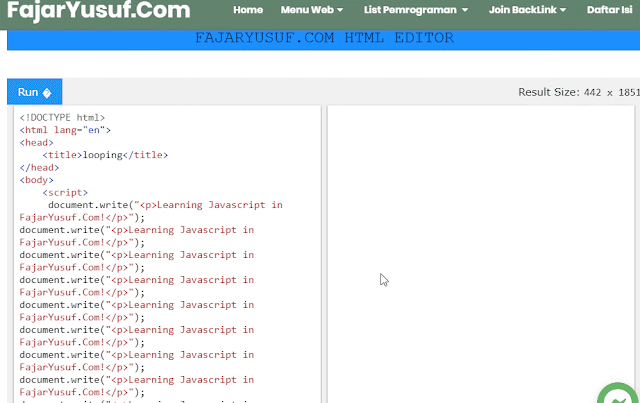click image to enlarge
The result:

Displays text without looping
Is it okay like this?

Yes, it's okay.

But ...

What if he'll want to show as much as 1000 times.

Definitely so tired of typing 1000 times.

Therefore, we must use a loop or loops.

Iteration will help us execute code repeatedly, no matter how much we want.

There are five forms of looping in JavaScript. In general, the loop is split in two.

Namely: counted loop and uncounted loop .

The difference:

Counted Loop is a looping clear and is certainly a lot of recurrence.
While Uncounted Loop, a repetition is not clear how many times he had to repeat.
Recurrence included in Counted Loop:

• looping For
• looping foreach
• looping Recurrence

Recurrence included in Uncounted Loop:

• looping While
• looping Do/While

Let's discuss them one by one…

## Looping in Javascript

The for loop is included in the counted looping the loop, because it is clear how many times he will repeat.

It looks like this:
``````for(let i = 0; i < 10; i++){
document.write("<p>Perulangan ke-" + i + "</p>")
}``````

complete coding examples:
``````<!DOCTYPE html>
<html lang="en">
<title>looping</title>
<body>
<script>
for(let i = 0; i < 10; i++){
document.write("<p>Perulangan ke-" + i + "</p>")
}
</script>
</body>
</html>
```

```
Noteworthy are the conditions that exist in parentheses after the word for.

These conditions will determine:

1. The count will start from 0 (i = 0);
2. The count up how many? Until i <10;
3. Then in each iteration i will increase +1 (i ++).
4. The variable i in a for loop serves to store the count value.

So every iteration i will always do its value increased by one. Because we determine in part i ++.

This results in the output: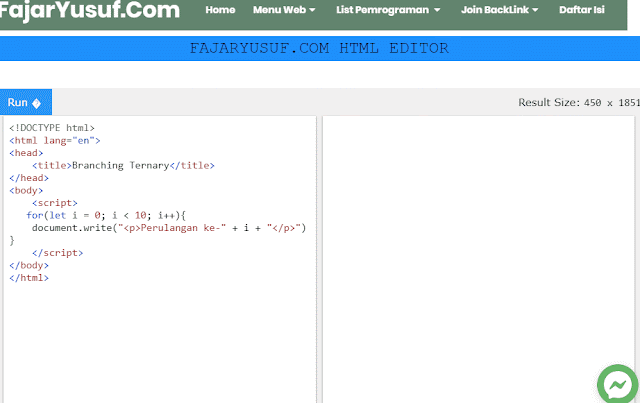click image to enlarge

Is the variable name should always i?

Not.

We can also use other names.

For example:

``````for(counter = 0; counter < 50; counter+2){
document.write("<p>Loop number-"+counter+"</p>");
}
```

```
In that example, we do looping starts from zero 0. Then in each iteration counter variable's value will plus 2 (counter + 2).

How about a recurrence counter starts from the larger number to which to the smallest?

This usually we make when trying to count down ...

How easy.

We stayed the contents of the counter value with the greatest value.

For example we will start to count from 10 to 0.

Then the counter value, we fill it first with 10.

Then the comparison condition, we give counter> 0. This means that the loop will be performed during the counter value is greater than 0.

Then we subtract (-1 ) the value of the counter at each iteration (counter--).

``````for(counter = 10; counter > 0; counter--){
document.write("<p>Looping number-"+counter+"</p>");
}
```

```
Coding details:
``````<!DOCTYPE html>
<html lang="en">
<title>looping</title>
<body>
<script>
for(counter = 10; counter > 0; counter--){
document.write("<p>Looping number-"+counter+"</p>");
}
</script>
</body>
</html>
```

```
Results: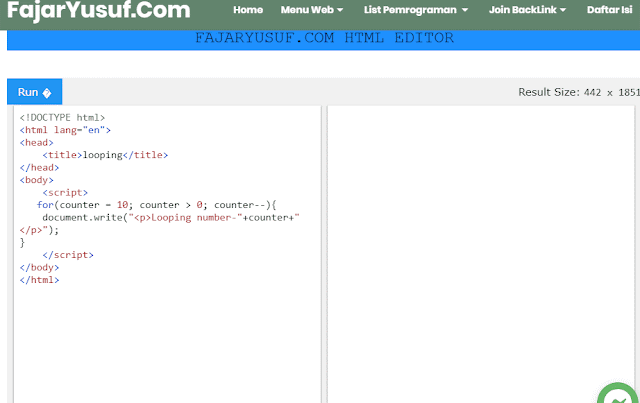click image to enlarge

Why not to zero (0)?

Due to the condition that we give counter> 0. If the counter value is 0, then this condition will become false.

Unless we use larger carriers is equal to (> =), then if the counter value is 0, the condition will be true.

## looping Whilein Javascript

While loops are loops that are included in uncounted loop iteration.

While loops also can be counted looping the loop by providing a counter in it.

To understand this looping ...

... let us look at an example:

``````<!DOCTYPE html>
<html lang="en">
<title>looping</title>
<body>
<script>
var repeat = confirm("Do you want to repeat?");
var counter = 0;
while(repeat){
var answer = confirm("Do you want to repeat?")
counter++;
repeat = false;
}
}
document.write("Repeat has been done as much as "+ counter +" times");
</script>
</body>
</html>
```

```
Can be simplified into:
``````<!DOCTYPE html>
<html lang="en">
<title>looping</title>
<body>
<script>
var repeat = confirm("Do you want to repeat?");
var counter = 0;
while(repeat){
counter++;
repeat = confirm("Do you want to repeat?");
}
document.write("Repeat has been done as much as "+ counter +" times");
</script>
</body>
</html>
```

```
The result:click image to enlarge
Try to note the code block while:

``````while(ulangi){
counter++;
repeat = confirm("Do you want to repeat?");
}
```

```
In the code block ... The loop will occur during repeat variable value is true.

Then we use the function confirm () to display a confirmation dialog.

As long as we choose Ok in the confirmation dialog, then the variable will repeat continuously evaluates to true.

But if we choose Cancel, then it will repeat variable is false.

When repeat variable is false, then the loop will be discontinued.

## Looping Do / While in Javascript

Recurrence do / while same as while loops.

The difference:

Repetition do / while loop will looping 1 times first, then check the condition that is in brackets while.

It looks like this:
``````do {
//the code block that will be repeated
} while (<condition>);
```

```
So the difference is:

Recurrence do / while loop will check the condition behind (after repeat), while while checks the condition before or early (before repeating).

Let us look at an example:

``````<!DOCTYPE html>
<html lang="en">
<title>looping</title>
<body>
<script>
var repeat = confirm("Do you want to repeat?");;
var counter = 0;
do {
counter++;
repeat = confirm("Do you want to repeat?");
} while(repeat)
document.write("Repeat has been done as much as "+ counter +" times");
</script>
</body>
</html>
```

```click image to enlarge
The same example as an example in the while loop.

When the first iteration, try to cancel its recurrence by choosing Cancel.

So the result:

## looping foreach in Javascript

Foreach iteration is usually used to print an item in the array.

This loop is included in counted looping the loop, because the number of recurrence will determined by the length of the array.

There are two ways to use foreach loops in Javascript:

1. Uses for the operator in;
2. Uses method forEach ().

Here is the form of the loop "foreach" without the use of carriers in:

``````<!DOCTYPE html>
<html lang="en">
<title>looping</title>
<body>
<script>
var languages = ["Javascript", "HTML", "CSS", "Typescript"];
for(i = 0; i < languages.length; i++){
document.write(i+". "+ languages[i] + "<br/>");
}
</script>
</body>
</html>
```

```
This loop can be made simpler by using the operator in this way:

``````<!DOCTYPE html>
<html lang="en">
<title>looping</title>
<body>
<script>
var languages = ["Javascript", "HTML", "CSS", "Typescript"];
for(i in languages){
document.write(i+". "+ languages[i] + "<br/>");
}
</script>
</body>
</html>
```

```
The result: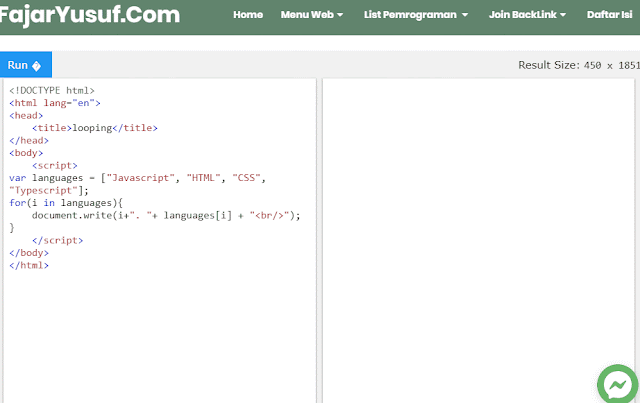click image to enlarge

The second way makes repeated foreach method is by using forEach () on the array.

Example:

``````<!DOCTYPE html>
<html lang="en">
<title>looping</title>
<body>
<script>
// we have an array like the following
var days = ["Monday", "Tuesday", "Wednesday", "Thursday", "Friday", "Saturday", "Sunday"];
// Then we show all days
// by using the foreach method
days.forEach(function(day){
document.write("<p>" + day + "</p>");
});
</script>
</body>
</html>
```

```
Method forEach () has a parameter such as a callback function. Actually, we can also use the arrow function like this:

``````<!DOCTYPE html>
<html lang="en">
<title>looping</title>
<body>
<script>
// we have an array like the following
var days = ["Monday", "Tuesday", "Wednesday", "Thursday", "Friday", "Saturday", "Sunday"];
// Then we show all days
// by using the foreach method
days.forEach((day) => {
document.write("<p>" + day + "</p>");
});
</script>
</body>
</html>
```

```
The result: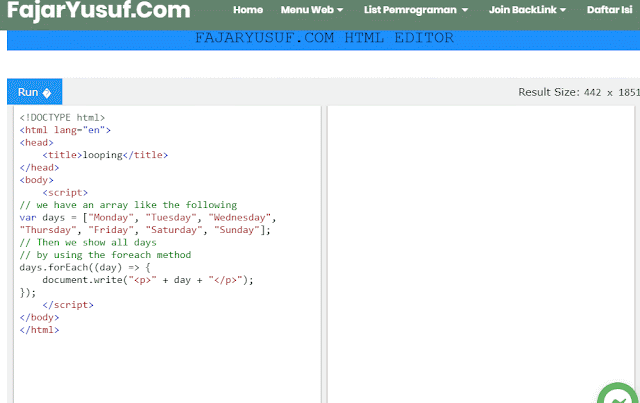click image to enlarge

## Looping Method with repeat ()

Iteration with the method or function repeat () included in the counted loop iteration.

This particular function is used to repeat a text (string).

You could say:

This is a short code of a for loop.

If we use a for loop:

``````<!DOCTYPE html>
<html lang="en">
<title>looping</title>
<body>
<script>
for( let i = 0; i < 100; i++){
document.write("Repeat this sentence!");
}
</script>
</body>
</html>
```

```
When we use the repeat function ():
``````<!DOCTYPE html>
<html lang="en">
<title>looping</title>
<body>
<script>
document.write("Repeat this sentence!".repeat(100));
</script>
</body>
</html>
```

```
The result:

## Looping Nested

In the block iteration, we also can make the loop.

This is called a nested loop or nested repetition or recurrence in looping.

Let us look at an example:

``````<!DOCTYPE html>
<html lang="en">
<title>looping</title>
<body>
<script>
for(let i = 0; i < 10; i++){
for(let j = 0; j < 10; j++){
document.write("<p>Looping number-" + i + "," + j + "</p>");
}
}
</script>
</body>
</html>
```

```
The result:click image to enlarge

In the iteration, we use two loops for.

The first iteration using the variable i as a counter, while the second iteration uses a variable j as a counter.

Another example:

``````<!DOCTYPE html>
<html lang="en">
<title>looping</title>
<body>
<script>
var repeat = confirm("Do you want to repeat?");
var counter = 0;
while (repeat) {
counter++;
var asterik= "*".repeat(counter) + "<br>";
document.write(counter + ": " + asterik);
repeat = confirm("Do you want to repeat?");
}
</script>
</body>
</html>
```

```click image to enlarge
The result:

Later, we will use a lot of nested loops when working with two-dimensional arrays.

We can make as much as possible looping in another iteration.

However, we need to pay attention to the following:
The more looping, then the computer will be longer to process.
Of course this will make the website or our applications run slowly.
Therefore, use looping wisely